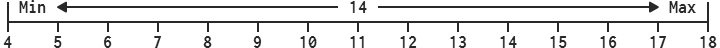# Range

Edit on GitHub
#include <Range.h>
SReturnNameParameters
Range (int32 value = 0)
Range (int32 minimum, int32 maximum)
int32 GetRange (void) const
void SetRange (int32 value)
void SetRange (int32 minimum, int32 maximum)
int32 GetRandom (void) const
bool Contains (int32 value, bool inclusive = true) const
bool operator == (const Range& range) const
bool operator != (const Range& range) const
int32 Min
int32 Max

## Description

Represents a range of two numbers defined by Min and Max with integer precision. Each component can be manipulated directly through the exposed property values. The GetRange function returns the difference between Min and Max while SetRange sets Min and Max to the specified parameters.

The Range class also supports an additional set of functionality including GetRandom, which returns a pseudorandom integer between [Min, Max) and Contains, which returns whether or not a value is between Min and Max.## Constructors

```

Range
(int32 value = 0)

Range
(int32 minimum,
int32 maximum)

```

Constructs a range with both Min and Max set to value or minimum and maximum.

## Functions

```

int32
GetRange
(void) const

```

Returns the difference between Min and Max as an integer.

```

void
SetRange
(int32 value)

void
SetRange
(int32 minimum,
int32 maximum)

```

Sets both Min and Max to value or minimum and maximum.

```

int32
GetRandom
(void) const

```

Returns a pseudorandom integer between Min (inclusive) and Max (exclusive). This function works similarly for both negative and positive inputs, however, if Max is less than or equal to Min then Min is returned. This function can be used for such purposes as generating input delays and randomizing sleep values, but distribution is not guaranteed.

Internally, this function uses a self-contained LCG-glibc algorithm, making it unsuitable for cryptographic purposes. Being self-contained means that this function does not depend on the compiler and thus, behaves more consistently across all platforms. Additionally, a separate state is used for each instance of this class, seeded at construction time using the current time.

```

bool
Contains
(int32 value,
bool inclusive = true) const

```

Returns true if value is between Min and Max. If inclusive is false, this function returns true only if value is entirely between Min and Max without being equal to either.

## Operators

```

bool
operator ==
(const Range& range) const

bool
operator !=
(const Range& range) const

```

Performs equality comparison on each component.

## Properties

```

int32
Min

int32
Max

```

Provides direct access to the minimum and maximum components of this range.

# Examples

``````// C++
#include <Robot.h>
ROBOT_NS_USE_ALL;

int main (void)
{
Range r1, r2 (6, 24);
// r1 now equals (4, 18)
r1.Min = 4; r1.Max = 18;

r1.GetRange (); // 14
r2.GetRange (); // 18
r1.GetRandom(); // Between [4, 18)
r2.GetRandom(); // Between [6, 24)

r1.Contains (3, false); // False
r1.Contains (4, false); // False
r1.Contains (4, true ); // True
r1.Contains (5, true ); // True

// r2 becomes (4, 18)
r2.SetRange (4, 18);

r1 == r2; // True
r1 != r2; // False
return 0;
}
``````
``````// Node
var robot = require ("robot-js");

var r1 = robot.Range ();
var r2 = robot.Range (6, 24);
// r1 now equals (4, 18)
r1.min = 4; r1.max = 18;

r1.getRange (); // 14
r2.getRange (); // 18
r1.getRandom(); // Between [4, 18)
r2.getRandom(); // Between [6, 24)

r1.contains (3, false); // False
r1.contains (4, false); // False
r1.contains (4, true ); // True
r1.contains (5, true ); // True

// r2 becomes (4, 18)
r2.setRange (4, 18);

r1.eq (r2); // True
r1.ne (r2); // False

// Range construction
r1 = robot.Range (r2);
r1 = robot.Range
({
min: 100,
max: 200
});
``````